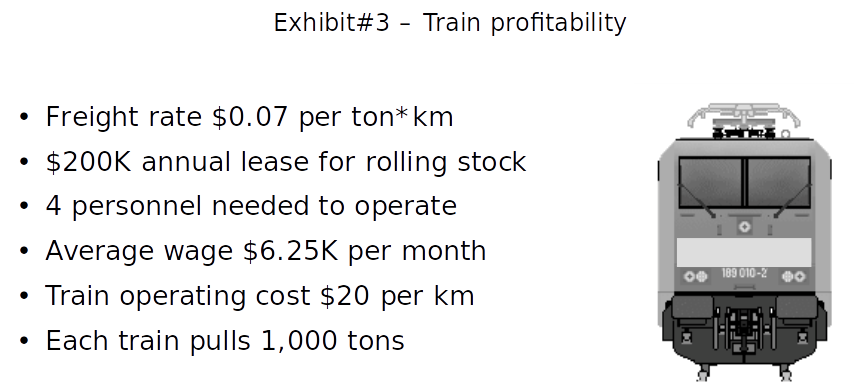# Question Breakeven

Bain
New answer on Aug 31, 2020
1.8 k Views

Hi !

Could you help me please with this question?, I can't get the correct answer that is B.

The average train for TrainCo carries 23 M km x tons per year, however, volume varies by line and the profitability for some of them might be negative. Given the data on Exhibit#3, which is the breakeven utilization for a train to be profitable?

(a) 7 M km x tons

(b) 10 M km x tons

(c) 12 M km x tons

(d) 23 M km x tons(edited)

• Date ascending
• Date descending

Plenty of people are rejected due to this breakeven question type. This is super easy with this structure ^^

STRUCTURE RULES
Structure the breakeven equation first. Define the breakeven metric. Calculate each component by translating the prompt. Do mental math shortcut and math priority

Breakeven structure
At breakeven,
Profit = 0
Revenu – cost = 0

Revenu structure
Revenue = Freight rate per ton*km x #km x #ton/train
Revenue = 0.07 x #km x 1000
= 70 x #km

Cost structure
Cost = Lease cost + Labor cost + Variable cost

• Lease cost = 200K per year
• Labor cost = #personnel x average wage per month x #month = 6.25K x 4 x 12 = 25K x 12 (mental math priority) =25K x 4 x 3 (mental math shortcut) =300K per year
• Variable cost = operating cost per km x #km = 20 x #km

Cost = 200K + 300K + 20 x #km
= 500K + 20 x #km

Breakeven resolution in km per year
Revenu - Cost = 0
70 x #km - 500K + 20 x #km = 0
(70-20) x #km = 500K
#km = 500K / 50
#km = 10 K per year

Breakeven resolution in km x tons per year
To break even the train need to achieve 10K km
Each train pull 1 000 tons
To break even the train need to achieve 10K km * 1 000 tons = 10K*1K = 10M km x tons

STRUCTURE FORMULAS
At breakeven, Profit = 0.
25 is a mental math priority
12 has as mental math shortcut 2 x 6 and 3 x 4

Upvote if it is useful ;)

(edited)